# 2 3 Solving MultiStep Equations Term A number

• Slides: 202. 3 Solving Multi-Step Equations: Term: A number, a variable or a product or quotient of numbers and variables that is added or subtracted in an algebraic expression. Combining like Terms: Simplify the terms with the same variable using arithmetic.GOAL: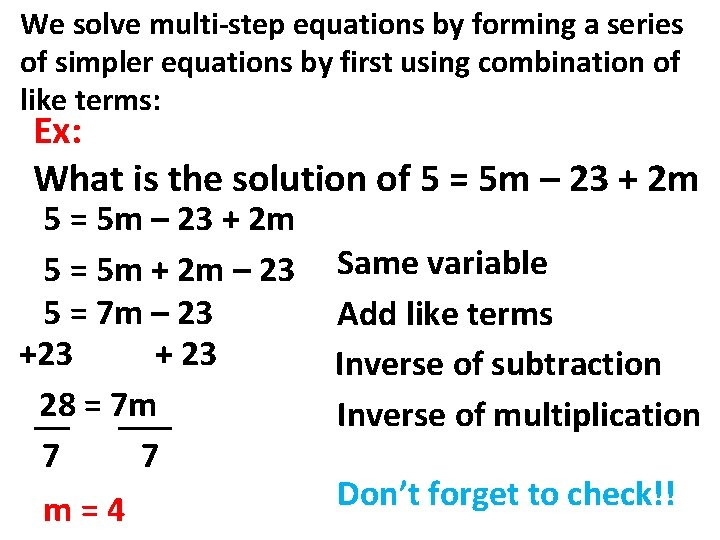We solve multi-step equations by forming a series of simpler equations by first using combination of like terms: Ex: What is the solution of 5 = 5 m – 23 + 2 m 5 = 5 m + 2 m – 23 5 = 7 m – 23 + 23 28 = 7 m __ ___ 7 7 m=4 Same variable Add like terms Inverse of subtraction Inverse of multiplication Don’t forget to check!!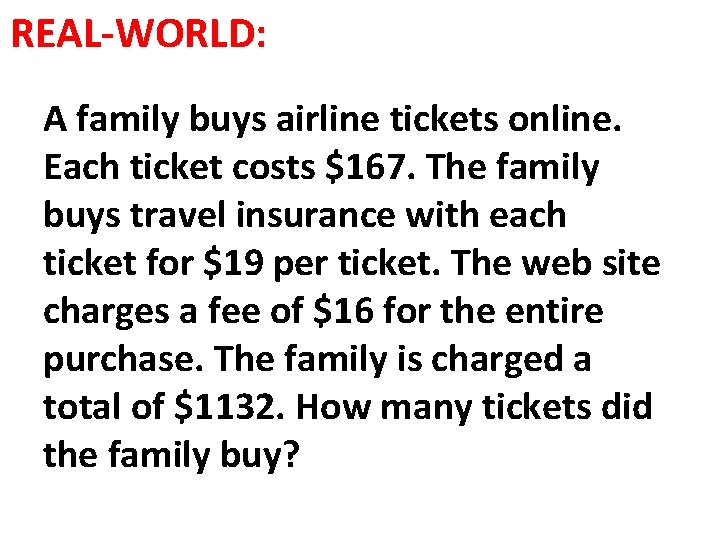REAL-WORLD: A family buys airline tickets online. Each ticket costs \$167. The family buys travel insurance with each ticket for \$19 per ticket. The web site charges a fee of \$16 for the entire purchase. The family is charged a total of \$1132. How many tickets did the family buy?SOLUTION: Using the given info we have: Each ticket (x) costs \$167 167 x Insurance for each ticket is \$19 19 x One time charge of \$16 +16 The family paid = \$1132 167 x + 19 x + 16 = 1132167 x + 19 x + 16 = 1132 186 x + 16 = 1132 Like terms 186 x + 16 -16 = 1132 -16 Inverse of Add 186 x = 1116 Like terms 186 x /186 = 1116 /186 Inverse of mult. x = 6 TicketsYOU TRY IT: What is the solution of 11 x – 8 – 6 x = 22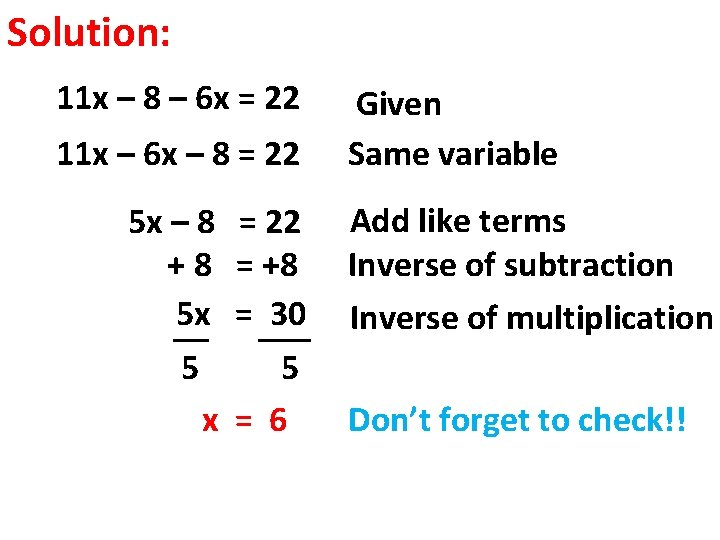Solution: 11 x – 8 – 6 x = 22 11 x – 6 x – 8 = 22 5 x – 8 +8 5 x __ 5 x = 22 = +8 = ___ 30 5 = 6 Given Same variable Add like terms Inverse of subtraction Inverse of multiplication Don’t forget to check!!Solving equations with Distributive Property: Ex: What is the solution of – 8 (2 x -1)= 36 – 8(2 x) – 8(-1)= 36 Distributive – 8 – 16 x + 8 = 36 Multiplication – 8 Inverse of addition –_____ 16 x = ____ 28 Inverse of multiplication – 16 x = - 28/16 - 7/4 Don’t forget to check!!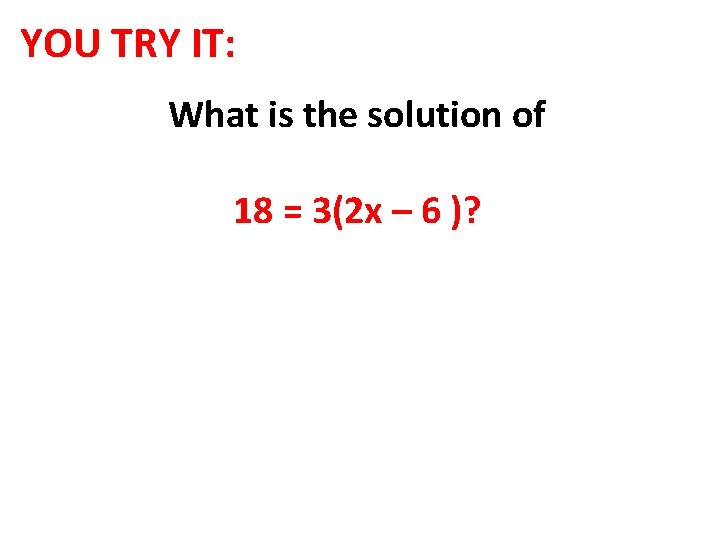YOU TRY IT: What is the solution of 18 = 3(2 x – 6 )?Solution: 18 = 3(2 x – 6) 18 = 3(2 x) + 3(-6) 18 = 6 x – 18 +18 36 6 x __ = ___ 6 6 x=6 Given Distributive 3 Multiplication Inverse of subtraction Inverse of multiplication Don’t forget to check!!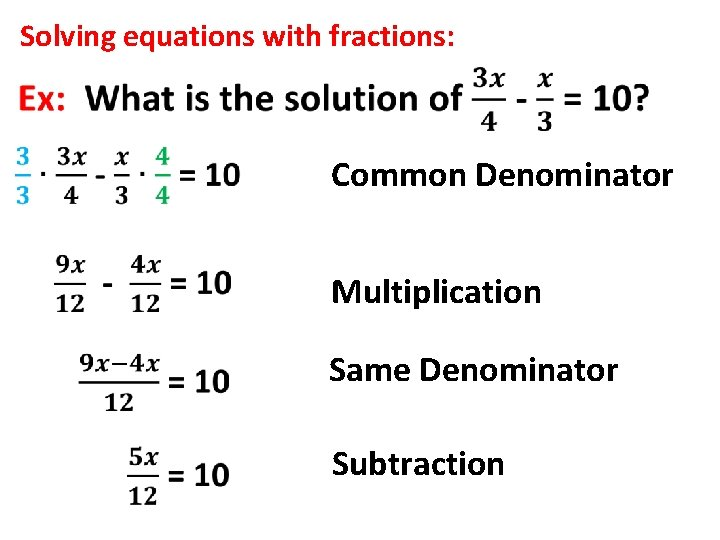Solving equations with fractions: Common Denominator Multiplication Same Denominator Subtraction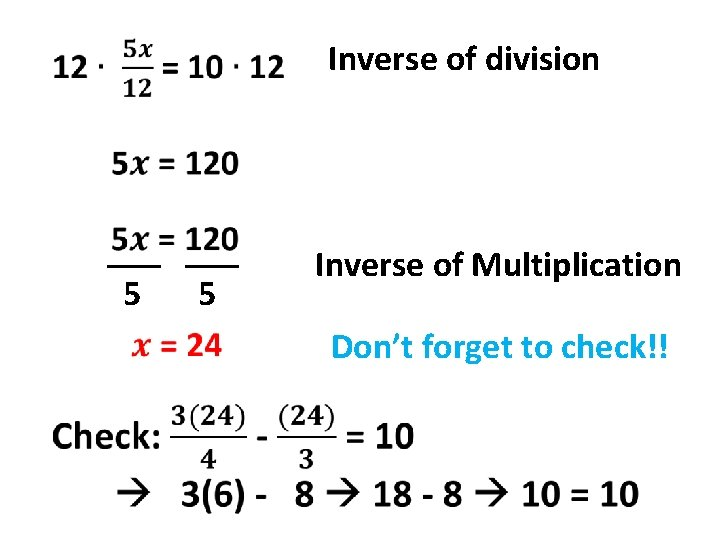Inverse of division ___ 5 5 Inverse of Multiplication Don’t forget to check!!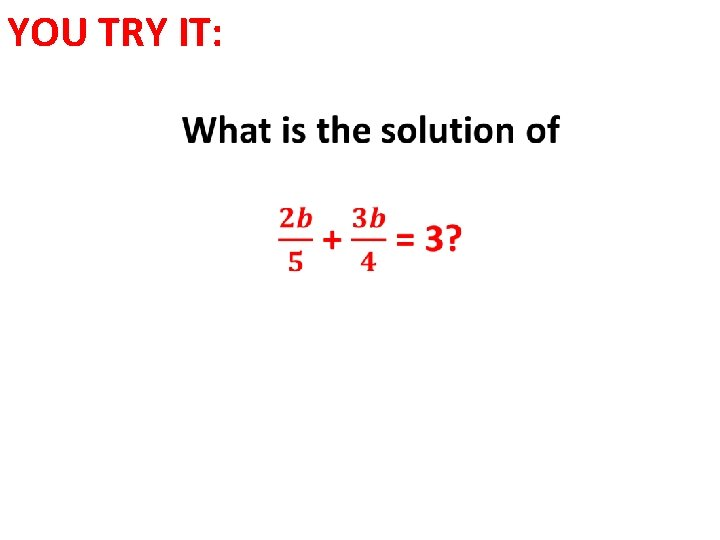YOU TRY IT: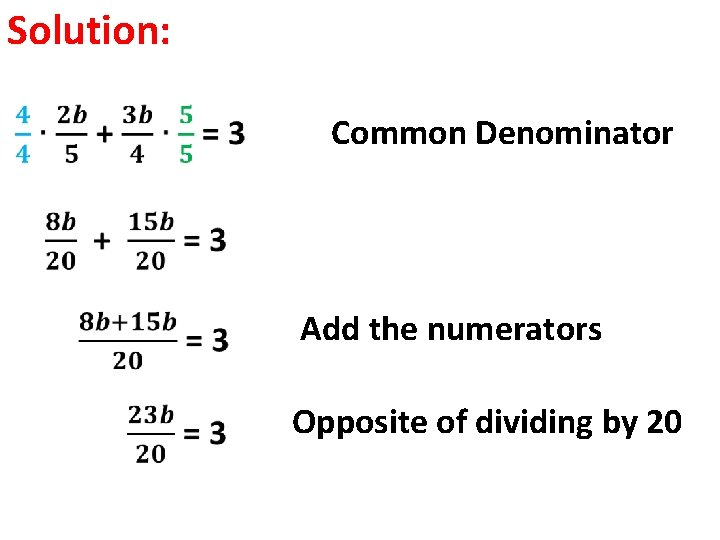Solution: Common Denominator Add the numerators Opposite of dividing by 20Solution: Multiply by 20 both sides 23 b = 60 Opposite of multiplying by 23 Don’t forget to Check!Plug in our answer We never divide fractions, We keep, change and flip!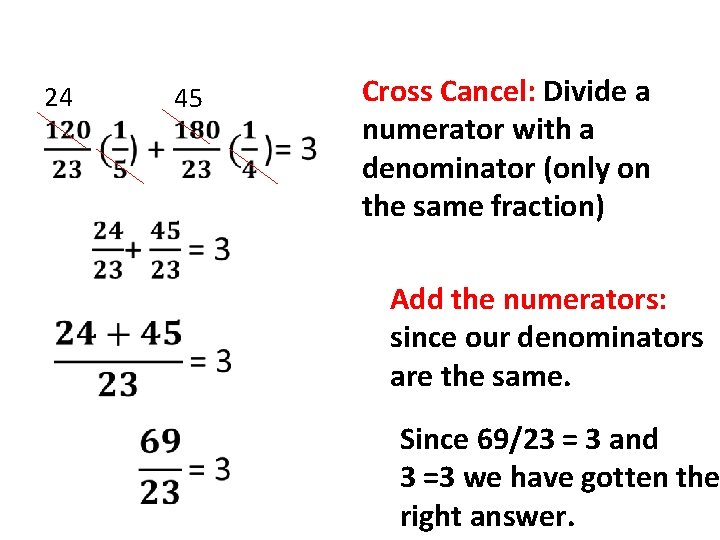24 45 Cross Cancel: Divide a numerator with a denominator (only on the same fraction) Add the numerators: since our denominators are the same. Since 69/23 = 3 and 3 =3 we have gotten the right answer.VIDEOS: Multi-Step Equations Multi-Step http: //www. khanacademy. org/math/algebra/ratio nal-expressions/solving-rational-equations/v/ex-1 multi-step-equation http: //www. khanacademy. org/math/algebra/solvi ng-linear-equations-andinequalities/complicated_equations/v/ex-2 -multistep-equationClass Work: Pages: 97 -100 Problems: As many as you need to master the concepts.